Search by Topic

Resources tagged with 2D representations of 3D shapes similar to Construct the Solar System:

Filter by: Content type:
Age range:
Challenge level:

There are 28 results

Broad Topics > 3D Geometry, Shape and Space > 2D representations of 3D shapes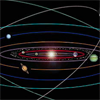Construct the Solar System

Age 14 to 18 Challenge Level:

Make an accurate diagram of the solar system and explore the concept of a grand conjunction.Perfect Eclipse

Age 14 to 16 Challenge Level:

Use trigonometry to determine whether solar eclipses on earth can be perfect.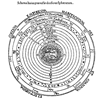Shaping the Universe II - the Solar System

Age 11 to 16

The second in a series of articles on visualising and modelling shapes in the history of astronomy.Shaping the Universe I - Planet Earth

Age 11 to 16

This article explores ths history of theories about the shape of our planet. It is the first in a series of articles looking at the significance of geometric shapes in the history of astronomy.Geometry and Gravity 1

Age 11 to 18

This article (the first of two) contains ideas for investigations. Space-time, the curvature of space and topology are introduced with some fascinating problems to explore.Perspective Drawing

Age 11 to 16 Challenge Level:

Explore the properties of perspective drawing.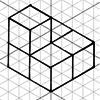Isometric Drawing

Age 11 to 14 Challenge Level:

Explore the properties of isometric drawings.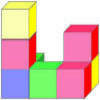Solids

Age 11 to 16 Challenge Level:

A task which depends on members of the group working collaboratively to reach a single goal.Chopped Dice

Age 14 to 16 Challenge Level:

Can you make a new type of fair die with 14 faces by shaving the corners off a cube?Age 14 to 18 Challenge Level:

How would you design the tiering of seats in a stadium so that all spectators have a good view?Bent Out of Shape

Age 14 to 18 Challenge Level:

An introduction to bond angle geometry.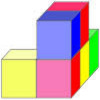The Solid

Age 11 to 16 Challenge Level:

A task which depends on members of the group working collaboratively to reach a single goal.Air Nets

Age 7 to 18 Challenge Level:

Can you visualise whether these nets fold up into 3D shapes? Watch the videos each time to see if you were correct.Which Face?

Age 14 to 16 Short Challenge Level:

Which faces are opposite each other when this net is folded into a cube?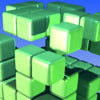The Perforated Cube

Age 14 to 16 Challenge Level:

A cube is made from smaller cubes, 5 by 5 by 5, then some of those cubes are removed. Can you make the specified shapes, and what is the most and least number of cubes required ?Moving Squares

Age 14 to 16 Challenge Level:

How can you represent the curvature of a cylinder on a flat piece of paper?Thinking 3D

Age 7 to 14

How can we as teachers begin to introduce 3D ideas to young children? Where do they start? How can we lay the foundations for a later enthusiasm for working in three dimensions?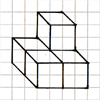Oblique Projection

Age 11 to 14 Challenge Level:

Explore the properties of oblique projection.Tennis

Age 11 to 14 Challenge Level:

A tennis ball is served from directly above the baseline (assume the ball travels in a straight line). What is the minimum height that the ball can be hit at to ensure it lands in the service area?Icosian Game

Age 11 to 14 Challenge Level:

This problem is about investigating whether it is possible to start at one vertex of a platonic solid and visit every other vertex once only returning to the vertex you started at.Cutting a Cube

Age 11 to 14 Challenge Level:

A half-cube is cut into two pieces by a plane through the long diagonal and at right angles to it. Can you draw a net of these pieces? Are they identical?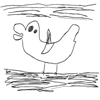The Development of Spatial and Geometric Thinking: 5 to 18

Age 5 to 16

This is the first article in a series which aim to provide some insight into the way spatial thinking develops in children, and draw on a range of reported research. The focus of this article is the. . . .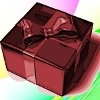Christmas Boxes

Age 11 to 14 Challenge Level:

Find all the ways to cut out a 'net' of six squares that can be folded into a cube.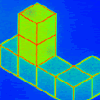Pupils' Recording or Pupils Recording

Age 5 to 14

This article, written for teachers, looks at the different kinds of recordings encountered in Primary Mathematics lessons and the importance of not jumping to conclusions!Take Ten

Age 11 to 14 Challenge Level:

Is it possible to remove ten unit cubes from a 3 by 3 by 3 cube so that the surface area of the remaining solid is the same as the surface area of the original?Nine Colours

Age 11 to 16 Challenge Level:

Can you use small coloured cubes to make a 3 by 3 by 3 cube so that each face of the bigger cube contains one of each colour?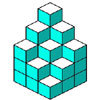Soma - So Good

Age 11 to 14 Challenge Level:

Can you mentally fit the 7 SOMA pieces together to make a cube? Can you do it in more than one way?The Spider and the Fly

Age 14 to 16 Challenge Level:

A spider is sitting in the middle of one of the smallest walls in a room and a fly is resting beside the window. What is the shortest distance the spider would have to crawl to catch the fly?